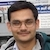# NAEST 2019 Screening Test: Torricelli's EquationBy

In screening round of National Anveshika Experimental Skills Test ( NAEST 2019 ), a question was asked on the fluid flowing out of two holes made at different heights of a container. In NAEST 2015 and NAEST 2017 questions were asked on the similar setup.

## Question from NAEST 2019

Question: Two pipes in the bottle are similar and both are horizontal. Choose the correct option(s)

1. The speed of water coming out from the upper pipe is more than the speed of water coming out from the lower pipe.
2. The water coming out from the two pipes takes equal time to reach the ground (red tray).
3. Water pressure at the level of the upper pipe is more than the water pressure at the level of the lower pipe.
4. The horizontal distance traveled by the water depends on the speed of the water coming out from the pipe as well as on the time it takes to reach the ground.

Solution: Consider a wide container having two identical holes 1 and 2, at heights $h_1$ and $h_2$ from the ground (see figure). The container is filled with water upto a height $y$ above the upper hole. The water starts flowing out of two holes in separate streams. These streams travels horizontal distances $x_1$ and $x_2$. How does $x_1$ and $x_2$ varies with $y$?Torricelli's law gives velocity of efflux at hole 1 and 2 as \begin{align} v_1 & =\sqrt{2gy} \\ v_2 & =\sqrt{2g (y+h_1-h_2)} \end{align} The velocity of water flowing out of the upper hole is less than that of water flowing out of the lower hole.

We assume that water stream takes parabolic path similar to that of a projectile thrown horizontally. The time taken by two streams to reach the ground are \begin{align} t_1&=\sqrt{2h_1/g} \\ t_2&=\sqrt{2h_2/g} \end{align} The upper stream takes more time to reach the ground. The horizontal distances travelled by two streams are \begin{align} x_1&=v_1 t_1=2\sqrt{h_1 y}, \\ x_2&=v_2 t_2=2\sqrt{h_2(y+h_1-h_2)} \end{align} The horizontal distances travelled by two streams are equal if $y=h_2$ i.e., when height of water level above the upper hole is equal to the height of the lower hole. The distance travelled in this condition is $x=2\sqrt{h_1 h_2}$.The variation of $x_1$ and $x_2$ with $y$ is shown in the figure. The stream from upper hole travels more distance if $y > h_2$. If $y < h_2$ then the stream from lower hole travels more distance.

## Question from NAEST 2017

Question 1: In the first part (bottle with one hole and cap open), the pressure $P_A$ at A (in the liquid) and the atmospheric pressure $P_0$ have relation

1. $P_A < P_0$
2. $P_A = P_0$
3. $P_A > P_0$

Answer: When cap is open, the pressure above the liquid surface is the atmospheric pressure $P_0$. If point A is at a depth $h$ then pressure at the point A (in the liquid) is \begin{align} P_A=P_0+\rho g h > P_0 \end{align} Thus, option (C) is correct. This pressure difference forces the water to flow out through hole A. Note that we used hydrostatic conditions because flow speed inside the bottle is very small.

Question 2: In the second part (bottle with two holes and cap closed), the pressure $P_A$, $P_B$, $P_0$ (A, B in the liquid) have relation

1. $P_A < P_0$
2. $P_A = P_0$
3. $P_A > P_0$
4. $P_B > P_0$
5. $P_B = P_0$
6. $P_B > P_0$

Answer: Observe carefully that water flows out from the lower hole A and air enter inside through the upper hole B. You can see a channel of air bubbles flowing to the top of air bottle. The pressure at B is equal to the atmospheric pressure because air channel connect this point to the outside atmosphere i.e., $P_B=P_0$.

If point A is at a depth $h$ below B then the pressure at the point A (in the liquid) is \begin{align} P_A=P_0+\rho g h > P_0 \end{align} This pressure difference forces the water to flow out through hole A.

## Question from NAEST 2015

Question 1: The stream from the lower pipe covers the least distance. This is because

1. Pressure of water in the bottle at the lower pipe is least.
2. Pipe diameter of the lower pipe is smaller than the others.
3. Height of the lower pipe over the floor is smaller than for other pipes.
4. Pressure of water in the bottle at the lower pipe is largest.

Answer: Correct option is (C). The time of flight is smallest for the lower pipe due to its smaller height ($t=\sqrt{2h/g}$). The distance covered (range) is the product of time of flight and the flow speed ($x=vt$). The range is minimum for the stream from lower pipe due to smaller $t$ (even though its flow speed $v$ is maximum).